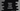# Python string rindex method explanation with example## Python string rindex method:

Python rindex method is a method defined in the string class. This method is used to get the rightmost index of a given substring in the string. We can pass the start and end indices to start and end the search in that string.

It is similar to another string method rfind. The only difference is that rindex throws ValueError if the substring is not found.

### Syntax of rindex:

The rindex method is defined as like below:

``s.rindex(substr[, start[,end]])``

Here,

• s is the string.
• substr is the substring to find in s.
• start is the start index of the search. This is an optional value and it takes 0 by default.
• end is the end index of the search. It is also an optional value. It takes the length of the string by default.

### Error thrown by rindex:

rindex throws ValueError if the substring is not found in that string.

### Example of rindex with characters:

Let’s try rindex with characters:

``````s = "Hello World !!"

print(s.rindex('r')) # 8
print(s.rindex('H')) # 0
print(s.rindex('o')) # 7``````

We are using rindex with different characters on the string s.

• The index of the last occurrence of r is 8
• The index of the last occurrence of H is 0
• The index of the last occurrence of o is 7

### Example of rindex with substrings:

The following examples uses substrings with rindex:

``````s = "Hello World Hello universe hello !!"

print(s.rindex('Hello'))  # 12
print(s.rindex('universe'))  # 18
print(s.rindex('ell'))  # 28``````
• Hello is appeared thrice. But, rindex is case-sensitive and it will ignore the last hello.
• universe is appeared only once.
• ell is picked from the last hello as rindex returns the index of the last occurrence.

### Example of rindex with start and end index:

We can pass optional start and end indices with the sub string to rindex. It will start the search from the start index and end it at end - 1 index.

``````s = "abcdabcdabcd"

print(s.rindex('a', 1)) # 8
print(s.rindex('a', 1, 5)) # 4
print(s.rindex('a', 4)) # 8``````

In the string s, a is appeared three times at index 0, 4 and 8.

• The first search starts from index 1 to the end of the string. So, it returns the last index of ‘a’ i.e. 8.
• The second search starts from index 1 and ends at index 4. So, it returns 4.
• The last one starts from index 4 to the end i.e. the result is 8.

### Example of ValueError:

As explained above, rindex throws ValueError if the substring is not found. For example,

``````s = "abcdabcdabcd"

print(s.rindex('i'))``````

i is not in s, so it will throw ValueError.

``````Traceback (most recent call last):
File "example.py", line 3, in <module>
print(s.rindex('i'))
``````s = "abcdabcdabcd"